[BACK]
 Computers, Materials & ContinuaDOI:10.32604/cmc.2022.020648Article

Distributed Secure Storage Scheme Based on Sharding Blockchain

1School of Computer Science and Mathematics, Fujian University of Technology, Fuzhou, 350118, China
2School of Computer & Communication Engineering, Changsha University of Science & Technology, Changsha, 410004, China
3School of Business, Nanjing University, Nanjing, 210093, China
4School of Computer, School of Software, School of Cyberspace Security, Engineering Research Center of Digital Forensics, Ministry of Education, Nanjing University of Information Science & Technology, Nanjing, 210044, China
5Department of Biomedical Engineering, University of Reading, RG6 6AY, UK
*Corresponding Author: Xiaofeng Yu. Email: xiaofengyu@nju.edu.cn
Received: 01 June 2021; Accepted: 17 July 2021

Abstract: Distributed storage can store data in multiple devices or servers to improve data security. However, in today's explosive growth of network data, traditional distributed storage scheme is faced with some severe challenges such as insufficient performance, data tampering, and data lose. A distributed storage scheme based on blockchain has been proposed to improve security and efficiency of traditional distributed storage. Under this scheme, the following improvements have been made in this paper. This paper first analyzes the problems faced by distributed storage. Then proposed to build a new distributed storage blockchain scheme with sharding blockchain. The proposed scheme realizes the partitioning of the network and nodes by means of blockchain sharding technology, which can improve the efficiency of data verification between nodes. In addition, this paper uses polynomial commitment to construct a new verifiable secret share scheme called PolyVSS. This new scheme is one of the foundations for building our improved distributed storage blockchain scheme. Compared with the previous scheme, our new scheme does not require a trusted third party and has some new features such as homomorphic and batch opening. The security of VSS can be further improved. Experimental comparisons show that the proposed scheme significantly reduces storage and communication costs.

Keywords: Blockchain; distributed storage; verifiable secret share; polynomial commitment

1  Introduction

Traditional centralized storage systems use centralized storage servers to store all data, which places high requirements on server performance, including reliability and security. At the same time, with the explosive growth of network data, centralized storage systems cannot satisfy the needs of large-scale applications. As a peer-to-peer storage method, distributed storage is gradually replacing traditional storage methods [1,2]. Distributed storage is to distribute data to multiple data storage servers to share the load to improve data security and storage efficiency. Nowadays, distributed storage has been widely used and favored by many companies. Common distributed storage systems, such as an efficient and scalable distributed file storage system called GFS proposed by Google.

However, distributed storage still has some problems in data security and system performance:

1)    Data security. Data security is always a hot topic. When network failure or equipment abnormality occurs, data may be lost. The user may lose part or all of the data. In addition, some malicious attackers will also steal or tamper with the stored data.

2)    Data management. Usually, the data is stored in different devices or servers. Different servers may have different data types, which is inconvenient for data management. In addition, because the update is not timely, the software version number may be different.

3)    Performance issues. The performance of distributed storage mechanisms is equally important. Such as capacity expansion and network optimization.

The combination of blockchain and distributed storage technology in the database provides a way to solve the above problem. The distributed storage system based on the blockchain can be used to securely store all kinds of data, and can be applied to fields such as smart grid, smart home, and Internet of Vehicles. As the underlying technology of Bitcoin, blockchain has received widespread attention due to its strong security characteristics . Blockchain was originally used to construct cryptocurrency. Because the blockchain has the characteristics of anti-tampering, openness and transparency, it was subsequently regarded as one of the methods to construct a secure data storage scheme . The blockchain itself is a distributed setting, but because of the Merkle tree structure used in data storage, it needs to pay more storage costs when dealing with large-scale applications.

Secret share combined with blockchain has some applications such as electronic voting, consensus algorithms, and P2P storage scheme . Such a scheme usually requires the participation of a Dealer, and we cannot guarantee that the Dealer is credible. This paper proposes an improved verifiable secret share scheme based on polynomial commitment without Dealer to replace the secret share scheme in distributed storage blockchain.

The specific contributions of this paper are as follows:

(1)   This paper proposes a verifiable secret share scheme based on polynomial commitment (PolyVSS, for short). Compared with the previous scheme, our new scheme does not require a trusted third party and has homomorphic characteristics.

(2)   Use PolyVSS to construct a distributed storage scheme based on blockchain. This scheme uses sharding technology to realize the partitioning of nodes and transactions. Experimental comparisons show that the proposed scheme can reduce storage and communication costs.

The structure of this paper is as follows. In Section 2, we introduce the related work of this paper. In Section 3, we first give the structure of a distributed storage blockchain based on PolyVSS. Section 4 introduces the proposed PolyVSS and analyzes its security. In Section 5, we analyzed the performance of the distributed storage blockchain and summarized in Section 6.

2  Related Work

2.1 Verifiable Secret Share

Secret share is one of the important research directions of modern cryptography. The earliest secret share scheme was proposed by Shamir. In their scheme, there is a dealer who is responsible for dividing a secret into n parts and distributing them to n members. After knowing any t or more shares (tn), these members can reconstruct the secret.

Due to the excessive trust given to the dealer, we cannot guarantee that the dealer will not have malicious behavior. To prevent the dealer from malicious behavior, verifiable secret share (VSS) is proposed . Verifiable secret share is based on secret share, adding a step of share verification. To put it simply, members verify the legitimacy of the secret distributed by the dealer. An important feature of VSS is unconditional privacy. This feature prevents the shared information from being obtained by a collection of members without permission. In addition to VSS, some practical variants of VSS schemes have been proposed, such as verifiable multi-secret share , non-interactive verifiable secret share, and public verifiable secret share.

Harin et al. [13,14] gave the formal definition of (n, t, n) secret share. In this scheme, n share-holders participate in sharing a master secret together, and everyone can randomly select a sub-secret and use an algorithm to generate sub-shares. Then using the homomorphic feature, each shareholder can combine all the sub-shares into the master share. Finally, the master share can be restored to the master secret through the reconstruction algorithm.

2.2 Polynomial Commitment

The concept of commitment is at the core of almost all modern cryptographic protocol constructions. In this case, making a commitment simply means that a participant in the protocol can choose a value from a certain (limited) set and commit to his choice so that he can no longer change his mind. However, he does not have to reveal his choice (although he may choose to reveal it at some point in the future). Cryptography commitment has been applied to the blockchain. Zerocoin  uses Pedersen commitment to bind a series of numbers s to Zerocoin z. The commitment C is as follows:

C=gshzmodp(1)

where p is unknown. Given the generators g and h, the user randomly selects the random numbers s and z, and the commitment C can be calculated. It is difficult to calculate the random numbers s and z when only knowing the commitment C, even if one of them is revealed. In addition to this, Kate et al.  proposed the first efficient polynomial commitment, which was subsequently used to construct a blockchain-based zero-knowledge proof protocol. Their scheme has the characteristics of a static accumulator. Next, we will introduce the construction of polynomial commitment:

The polynomial commitment scheme is constructed based on bilinear pairing. First, we use G=e,G,GT to represent the generation of bilinear groups (see Definition 6). The algorithm of polynomial commitment can be divided into four phases:

1)    Initialization phase:

This step mainly generates a public-private key pair pk,sk, where the public key is expressed aspk=G,g,gϑ,gϑ2,gϑn.The private key sk=ϑ cannot be used in the next steps.

2)    Commit phase:

Calculate the corresponding commitment C=gF(ϑ)G. Since the polynomial can be expressed as x=j=0deg(F)Fjxj,deg(F)t, the commitment can also be written as:

C=i=0deg(F)(gϑi)Fi(2)

3)    Open phase:

This step opens the committed polynomial C.

4)    Verify phase:

At this phase, the verifier first needs to verify the legitimacy of the commitment:

C=gF(ϑ)(3)

If the equation holds, the verification passes. Otherwise, it fails. Then output a triple α,F(α),ωα, where ωα=gfα(ϑ) is the witness in the index α.gfi(ϑ) satisfies:

fα(ϑ)=F(ϑ)F(α)ϑα(4)

Finally, verify the evaluation in the index α:

e(C,g)=e(ωα,gϑ/gα)e(g,g)F(α)(5)

If the equation holds, the verification passes. Otherwise, it fails.

Suppose there is an adversary Θ. The polynomial commitment satisfies the three characteristics of polynomial binding, evaluation binding, and computational hiding:

Polynomial Binding. We say that the polynomial commitment is polynomial binding if it is satisfied:

Pr[pkInitial(1k),(C,Fx,Fx)Θ(pk):VerifyPoly(PK,C,Fx)=1VerifyPoly(PK,C,Fx)=1FxFx]=ϵ(κ)(6)

Evaluation Binding. We say that the polynomial commitment is evaluation binding, if it is satisfied:

Pr[pkInitial(1k),(C,α,F(α),ωα,α,F(α),ωα)Θ(pk):VerifyPoly(pk,C,α,F(α),ωα)=1VerifyPoly(pk,C,α,F(α),ωα)=1FxFx]=ϵ(κ)(7)

Computational Hiding. Assuming there is an adversary Θ, given pk,C and iυ,F(iυ),ωFαυ. Where 1υdeg(F), and for each υ, the verify phase can be verified successfully. No adversary Θ can determine F(υ^) with non-negligible probability for any un-queried index υ^.

In addition, the polynomial commitment also satisfies strong correctness, the proof of which has been given in the paper .

3  The Proposed Distributed Storage Scheme Based on Sharding Blockchain

3.1 System Model of Distributed Storage Scheme Based on Blockchain

Before introducing the system model of DSB, we first introduce a few related notions. Let Bt denote the t-th block, Ht denote the hash value stored with the (i+1)th transaction, and hi=h(ψi). ψt=(hi1,h(Bi)), hi1 is the hash of the previous block. h and h are two hash functions respectively. The specific structure is shown in Fig. 1. The i-th block is hashed and stored together with the hash of the previous block.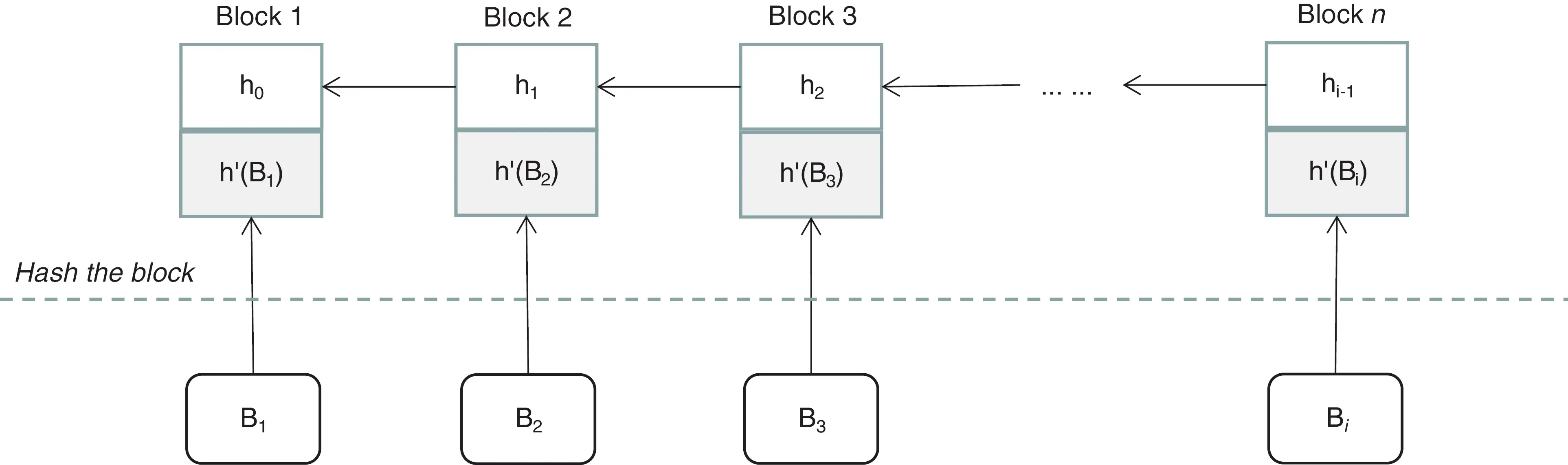Figure 1: Hash chain in distributed storage scheme based on blockchain

As we can see from the Fig. 1, the DSB scheme is to hash the entire block. Below we give the definition of DSB.

Definition 1 Distributed Storage Based on Blockchain (DSB). DSB consists of three phases.

First give a node partition:

χ={X,,Xnr+1}(8)

where n represents the total number of nodes.R=nr+1 indicates that the nodes are divided into R subsets of size r+1. The specific stages are as follows.

1)    Initial phase

For l[1,nr+1], the initialization algorithm randomly generates a key keyl(t).

2)    Encryption phase

There is an encryption algorithm denoted as ϕ, and the block can be encrypted with a key:

Ml(t)=ϕ(Bi,keyl(t))(9)

3)    Storage phase

Distribute and store Ml(t) among r+1 nodes in partition χ, and then use secret share algorithm to store keyl(t) and ψt.

3.2 The Structure of Distributed Storage Scheme Based on Blockchain

We constructed our storage scheme based on the blockchain, and introduce some of the corresponding concepts are related to the blockchain in this section . First, we will introduce the components of the framework of our scheme:

1)    Data management center (DMC): The data management center is responsible for sending data verification requests and distributing data to nodes in designated shard.

2)    Node: The node is responsible for the maintenance of the ledger and the verification of the data.

3)    Shard: With the help of blockchain sharding technology [20,21], the nodes in our scheme are randomly divided into a specified number of shards, and the number of nodes in each shard is the same.

4)    Blockchain database: The blockchain database is used to store data that has been verified by the nodes.

5)    P2P network: P2P networks have advantages in building distributed applications . Our scheme uses a distributed P2P network without central node, and a network is randomly established between nodes.

First, the DMC sends a request to the nodes. After receiving the request, each node runs PolyVSS three-phase algorithm to distribute and store data. Fig. 2 shows the structure of a sharding-based blockchain storage system (assuming that all nodes are divided into three shards). It should be noted that the structure is the same regardless of the number of shard. Each dashed box in the figure represents a shard, and each shard has the same number of nodes. The nodes in each shard are independent of each other, do not affect each other, and can communicate with each other when necessary. This can prevent malicious nodes in different shards from colluding with each other and prevent double-spending attacks. Of course, in order to prevent all malicious nodes from being divided into the same shard, we refer to the technique of the paper , so that the node allocation is completely random.

The number of nodes is not as many as possible. With reference to the practical Byzantine fault-tolerant algorithm, we generally limit the number of nodes in each shard to no more than 100. When the number of nodes exceeds 100, the efficiency of reaching consensus among nodes will become low. Of course we can increase the number of shard. In our scheme, there are a total of three shards and we assume that the number of nodes in each shard is 50.

Let the total number of nodes be Nub, F represents the number of shards, we have F=Nubr+1, where r+1 is the size of each shard. The specific scheme is given in the next section.

3.3 The Proposed Scheme Based on Sharding Blockchain

Our scheme is based on sharding blockchain, and can process multiple data in parallel, which theoretically improves the efficiency of data verification. Our scheme is divided into three phases: request phase, secret share phase and storage phase.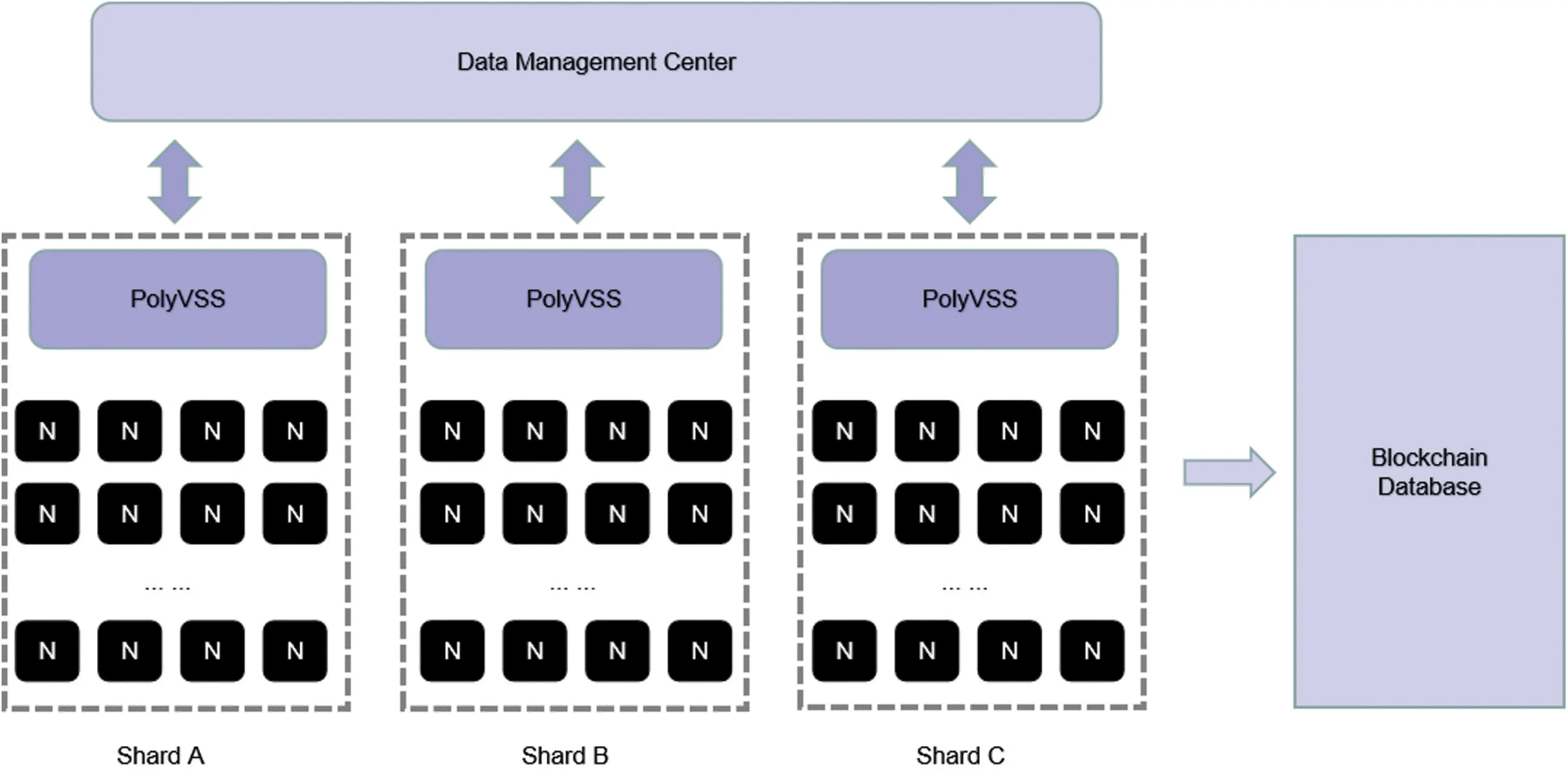Figure 2: Data storage scheme based on sharding blockchain

1)    Request phase

When a piece of data needs to be added to the chain, the Data Management Center (DMC) will send a request to all nodes in a shard.

2)    Data verification phase

Each node Ni independently selects a sub-secret Si, and the master secret can be expressed as

S=i=1nSi=S1++Sn(10)

For each sub-secret Si, Ni randomly selects a t-degree polynomial Fi(x), and the corresponding sub-secret is Fi(0)=Si.

Ni uses the Commit algorithm to generate the commitment C and broadcast it throughout the P2P network.

For j[1,n], Ni respectively calculates a witness wj and the sub-share:

sij=Fi(xj)(11)

and then sends j,Fi(xj),wj to other Ni in the network through a trusted channel.

After receiving j,Fi(xj),wj, each Ni starts to run the evaluation verification algorithm in the polynomial commitment.

After the verification is passed, all nodes accept the corresponding sub-secret, and use the Lagrange interpolation to restore the corresponding sub-secret.

3)    Data storage stage

After PolyVSS is executed, the verified data is uploaded to the blockchain. The specific process is shown in Fig. 3.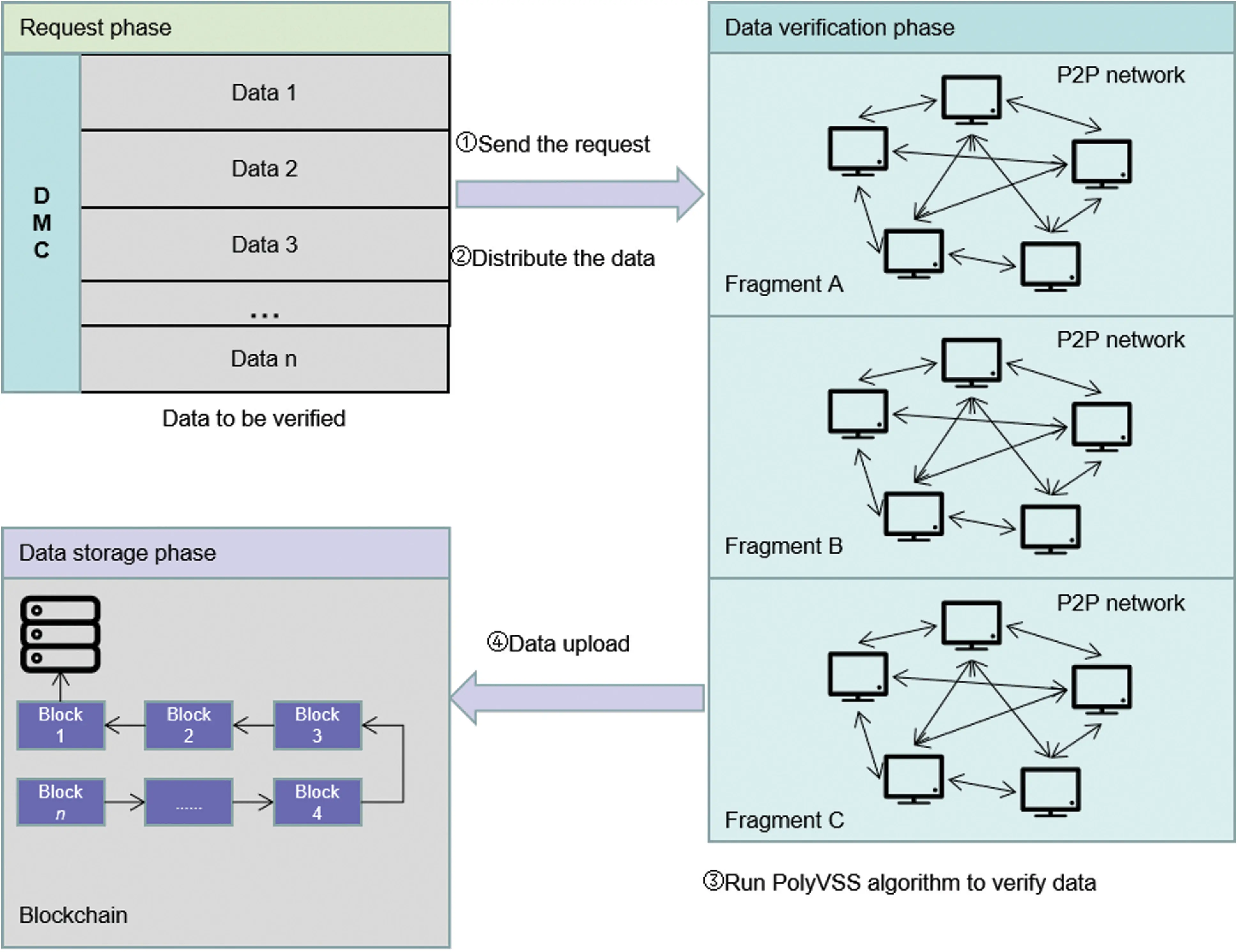Figure 3: Data creation, distribution, verification and storage process based on PolyVSS protocol

4  The Proposed Verifiable Secret Sharing Scheme Based on Polynomial Commitment

In this section, we will first introduce the formal definition of VSS and some cryptographic assumptions. Then, the specific construction is given. We also conduct security and performance analysis of the scheme.

4.1 Preliminary

First of all, we give the formal definition of VSS scheme and several security features that it needs to satisfy.

Definition 2 Verifiable secret share (VSS). A VSS scheme is divided into two phases:

Share phase: At the beginning of the phase, the Shareholder holds an input s, and the corresponding share can be calculated using s.

Reconstruction phase: With any t shares, users can use Lagrangian interpolation formulas to reconstruct the secret value.

To facilitate the description of the application later, in the following text we will use node instead of Shareholder. Usually, a VSS scheme needs to satisfy two security features: Secrecy and Correctness. Below we give their definitions.

Definition 3 Secrecy. The adversary cannot calculate the correct sharing s during the share phase.

Definition 4 Correctness. The reconstructed value should be equal to the shared secret s or every honest node will reach a result and accuse the node of maliciousness by outputting .

Some VSS schemes have introduced cryptographic commitment, such as Pedersen commitment with homomorphic characteristics. Cryptographic commitment generally consists of two phases: commit and open, which are respectively to commit and open the message. Polynomial commitment is also a kind of homomorphic commitment, which can be constructed based on discrete logarithm and Pedersen commitment. The polynomial commitment algorithm is based on the two traditional commitment algorithms, combined with the characteristics of the accumulator to add a verification algorithm. The existing research points of verifiable secret share scheme based on polynomial commitments are mainly in the scheme construction of asynchronous and synchronous models .

Here are a few cryptographic assumptions used for the security proof of our scheme.

Definition 5 Discrete Logarithm Assumption (DLA). Given a group G of generating elements g, G=G, and a random number ϑZP, the probability that gϑ is computed by ϑ is ϵκ for each adversary.

Definition 6 Bilinear Pairing. Let G1,G2 be the additive cyclic group of order p, GT is the multiplicative group of the same order, and e:G1,G2GT is expressed as a bilinear mapping.

Assuming MG1, NG2, α,βZp, the bilinear pairs satisfy three properties:

(1)   Bilinear: e(Mα,Nβ)=e(M,N)αβ;

(2)   Non-degenerate: There exists M and N satisfy e(M,N)1;

(3)   For any M and N, there exists an efficient algorithm that allows the result of e(M,N) to be derived in polynomial time (PPT).

4.2 The Proposed Scheme Based on Polynomial Commitment

Our scheme is an improvement on the (n, t, n) verifiable secret sharing scheme . In the (n, t, n) scheme, the first n represents n sub-shares, t represents the threshold, the knowledge of the threshold cryptography is used here, and the last n represents n participants. One advantage of such a scheme is that it does not require a trusted third party, which is not completely trusted. Scheme without a trusted third party can improve security.

On the basis of the previous scheme, polynomial commitment is introduced. Our scheme is divided into two phases: share phase and reconstruction phase. At the beginning of the scheme, the node runs the initial algorithm in the polynomial commitment, randomly selects a generator g, a random number αZp, and then generates a public key pk=g,g,gα,,gαt.

1)    Share phase

Master secret generation algorithm: Each node Pi independently chooses a sub-secret Si, the master secret can be expressed as S=i=1nSi=S1++Sn.

Share generation algorithm: For each sub-secret Si, Pi randomly selects a t-degree polynomial Fi(x), and the corresponding sub-secret is Fi(0)=Si. Then run the commit algorithm in the polynomial commitment to generate a commitment C=gF(α) and broadcast it throughout the P2P network. Forj[1,n], Pi calculates sub-shares sij=Fi(xj), a witness wj, and sends j,Fi(xj),wj to other Pi in the network. The master share can be expressed ass=i=1nsij.

Verification algorithm: After receiving j,Fi(xj),wj, each Pi starts to run the verify algorithm in the polynomial commitment. If the verification of a share holder Pi fails, other nodes will return an accusation message to oppose Pi. If more than t nodes accuse Pi, obviously, Pi is wrong and disqualified. On the contrary, Pi broadcasts the corresponding share and i,Fi(x),wi to the accusing party. If the revealed share fails to be verified again, then Pi is unqualified and the agreement ends, otherwise, each Pi accepts sij.

2)    Reconstruction phase:

In the reconstruction phase, when t+1 shared holders pass the verification algorithm, each Pi interpolation pair i,Fi(x) to determine Si=Fi(0), and then calculates the master secretS.

4.3 Analysis of the Proposed PolyVSS Scheme

4.3.1 Security Analysis

First, we give the adversary model. We consider a network P={P1,P2,,Pn} composed of n participants. Our adversary Θ is t-bounded and adaptive and can compromise and coordinate the actions of up to t of n parties. It can damage any party under any circumstances during the execution of the protocol, as long as the amount of damage is bounded by t.

Theorem 1: The proposed VSS scheme based on polynomial commitment satisfies correctness and secrecy.

Proof: We will prove that our scheme satisfies the correctness and secrecy features.

Correctness. Compared with other VSS schemes, our scheme does not have dealers. That is to say, in our scheme, we do not need to consider whether the dealer is honest. Suppose that the node uses the polynomial F(x) to share a secret s and remains honest throughout the execution of the sharing phase. Let C be the commitment sent to each node. Considering the strong correctness of the polynomial commitment, all honest nodes will get the correct share of the secret s consistent with C. Suppose a malicious node is allowed to broadcast its triplet i,Fi(x),wi, but the final verified value is not equal. Since polynomial commitment is computational binding, only honest nodes can reconstruct the secret.

Secrecy. The secrecy of our scheme comes from the hiding feature of polynomial commitment. Regardless of whether the node is malicious or honest, it is difficult for an adversary to obtain secret-related information. Suppose there is a t-bounded adversary Θ, which can obtain t messages i,Fi(x),wi. Since polynomial commitment is constructed based on discrete logarithms, it has hiding features. Below we first prove hiding.

Suppose there is an algorithm E constructed by adversary that can break the DLA. Let g,gϑ as an instance of the discrete logarithm problem that algorithm E needs to solve. Algorithm E randomly chooses a number ϑZP to generate a public key pk=G,g,gϑ,gϑ2,,gϑn to the adversary Θ. Algorithm E sets τ,ϕ(τ) as the index of polynomial ϕ(x) at index τ. Then suppose ϕ(0)=u, which is the answer to the DL instance, and use n+1 exponential evaluation to calculate gϕ(x), 0,gϑ and other selected pairs τ,gϕ(τ). Finally, E calculates the testimony τ,F(τ):

ωτ=(gϕ(ϑ)/gϕ(τ))1ϑτ(12)

And send pk and witness tuple τ,ϕ(τ),ωτ to the adversary Θ. Once the adversary Θ returns the polynomial ϕ(x), E returns the constant term ϕ(0) as the solution of the DLA instance.

It is easy to see that the success probability of solving the DLA instance is the same as the success probability of Θ, and the time required is larger than the time required by Θ by a small constant. That is, it is impossible to reconstruct the polynomial F(x) and the corresponding secret by only revealing such t messages.

4.3.2 PolyVSS Performance Analysis

This section compares the computational costs and functions of the six schemes in the four stages of parameter setting, reconstruction, verification, and recovery.

The polynomial commitment scheme given in Section 2.2 can only open and verify the evaluation of one index and is not suitable when multiple guidelines need to be opened. A batch polynomial commitment was proposed to open and verify the evaluation of multiple indexes. The batch polynomial commitment mainly modifies the verify phase. Let all the indexes τ to be opened form a set WZp, that is τW. W satisfies |W|<t. Algorithm output triples W,r(x),ωW, where ωW=gfw(α) is the witness of all indexes. h(x) is expressed as the remainder of F(x)iW(xi).fw(x) is expressed as:

fw(x)=F(x)h(x)iW(xi)(13)

Finally, the verifier verifies the correctness of the following equation:

e(C,g)=e(giW(xi),ωW)e(g,gh(x))(14)

With the aid of batch polynomial commitment, when n indexes need to be opened, the burden of witness calculation is reduced from n to 1.

We compared the computational cost and functions of several VSS schemes . The specific comparison is shown in Tab. 1, where n represents how many operations are done, and t can be represented as the number of nodes. The function comparison is shown in Tab. 2.

5  Performance Analysis of Our Proposed Distributed Storage Scheme

5.1 Security Analysis

Denial of service (DoS) attack is a method used to disrupt legitimate users’ access to the target network or website resources . Usually this is achieved by overloading a target with a large amount of traffic (usually a web server), or by sending malicious requests that cause the target resource to malfunction or completely collapse .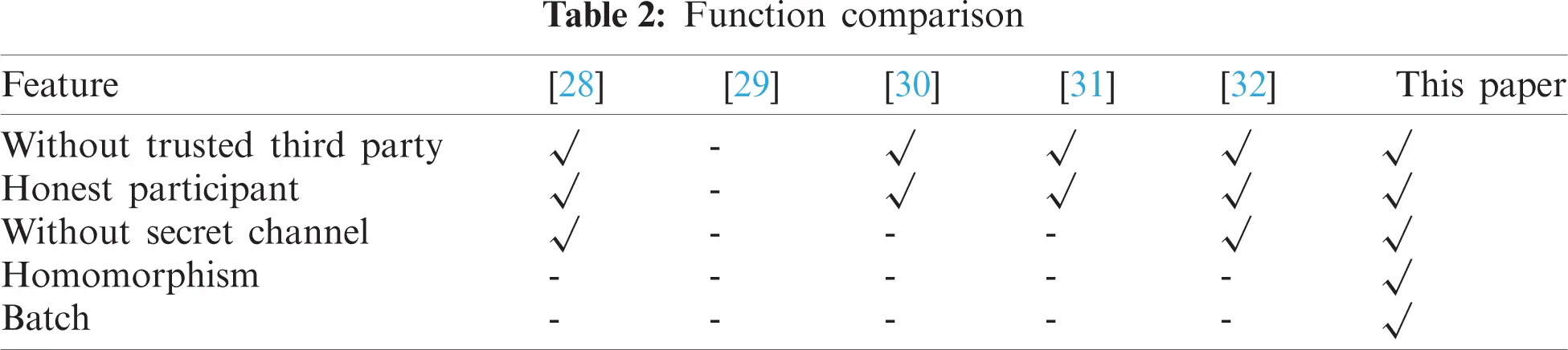Blockchain will also suffer from DoS attacks. In the traditional blockchain, when a node is attacked, it needs to visit other nodes (because each node stores the entire ledger) to recover local data. In our scheme, when a node in the network is attacked, the node can use the reconstruction algorithm of the PolyVSS scheme to recover the corresponding data by accessing other r+1 nodes. Therefore, our scheme can effectively deal with single point of failure.

Theorem 2: The proposed distributed storage scheme can reconstruct secret by accessing any r+1 nodes.

Proof: Since deg(F)t, the polynomial F(x) can be interpolated by accessing any r+1 nodes.

Below we analyze the cost of restoring communication. For convenience, we use DSB and LSS-DSB respectively to replace the name of the scheme in the paper . The data of the corresponding schemes are given in Tab. 3. We use symbols Stor to represent recovery communication cost, and symbols Com to represent storage cost.

The core of our scheme is the secret sharing scheme, which is also an important tool to achieve recovery. The Shamir secret share used in DSB is one of the most classic schemes. Local secret share is based on Shamir secret share, introducing two new concepts: global secret and local secret. Among them, information as global secret is more important than a local secret. Global secrets are maintained by all users, while local secrets are maintained by individuals. Unlike their two schemes, our scheme does not have a central party, such as the dealer in the Shamir's scheme. In addition, participants in our scheme will mutually verify the legality of share, thereby improving security.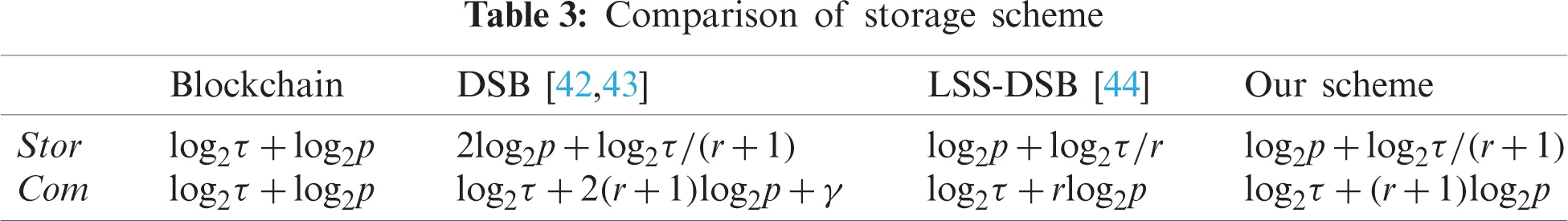Blockchain. Due to the characteristics of traditional blockchains, each node needs to store the entire ledger. When a single point of failure occurs, it is necessary to access all other nodes to restore all transaction data. Assuming BtFτ, ψtFp, where Fτ, Fp are two prime number domains, so the recovery communication cost is:

ComBlog2τ+log2p(15)

The symbol means proportional. Once the size of the prime number field is determined, the storage cost of the blockchain is fixed.

DSB. Nodes need to visit r+1 other subsets of nodes to recover all data in DSB. Assuming Ml(t)Fτr+1, keyl(t)Fp, ψtFp, so the recovery communication cost is:

ComDSB=log2τ+2(r+1)log2p+γ(16)

γ represents the additional cost of accessing other subsets and its value is fixed. Obviously, the recovery communication cost is related to r, and as r increases, the communication recovery cost also increases.

LSS-DSB. The node can recover the entire data by accessing r subsets locally. Compared with DSB, no additional recovery communication cost is required. The recovery communication cost is:

ComLSSDSB=log2τ+rlog2p(17)

Our scheme. In our scheme, the node also needs to access r+1 other nodes to recover data. The recovery communication cost is:

ComPolyVSSDSB=log2τ+(r+1)log2p(18)

Assumingp=2400, τ=240, the recovery communication cost is shown in Fig. 4. Our scheme is superior to DSB in terms of communication cost, similar to LSS-DSB.

5.2 Storage Analysis

In this section, we will compare the storage cost of several schemes when storing a transaction. The data of the corresponding schemes are given in Tab. 3, here is a brief analysis of several schemes.

Blockchain. In traditional blockchains based on Bitcoin, nodes usually store the entire transaction ledger. The storage overhead for each node of the blockchain to store a transaction is:

StorB=log2τ+log2p(19)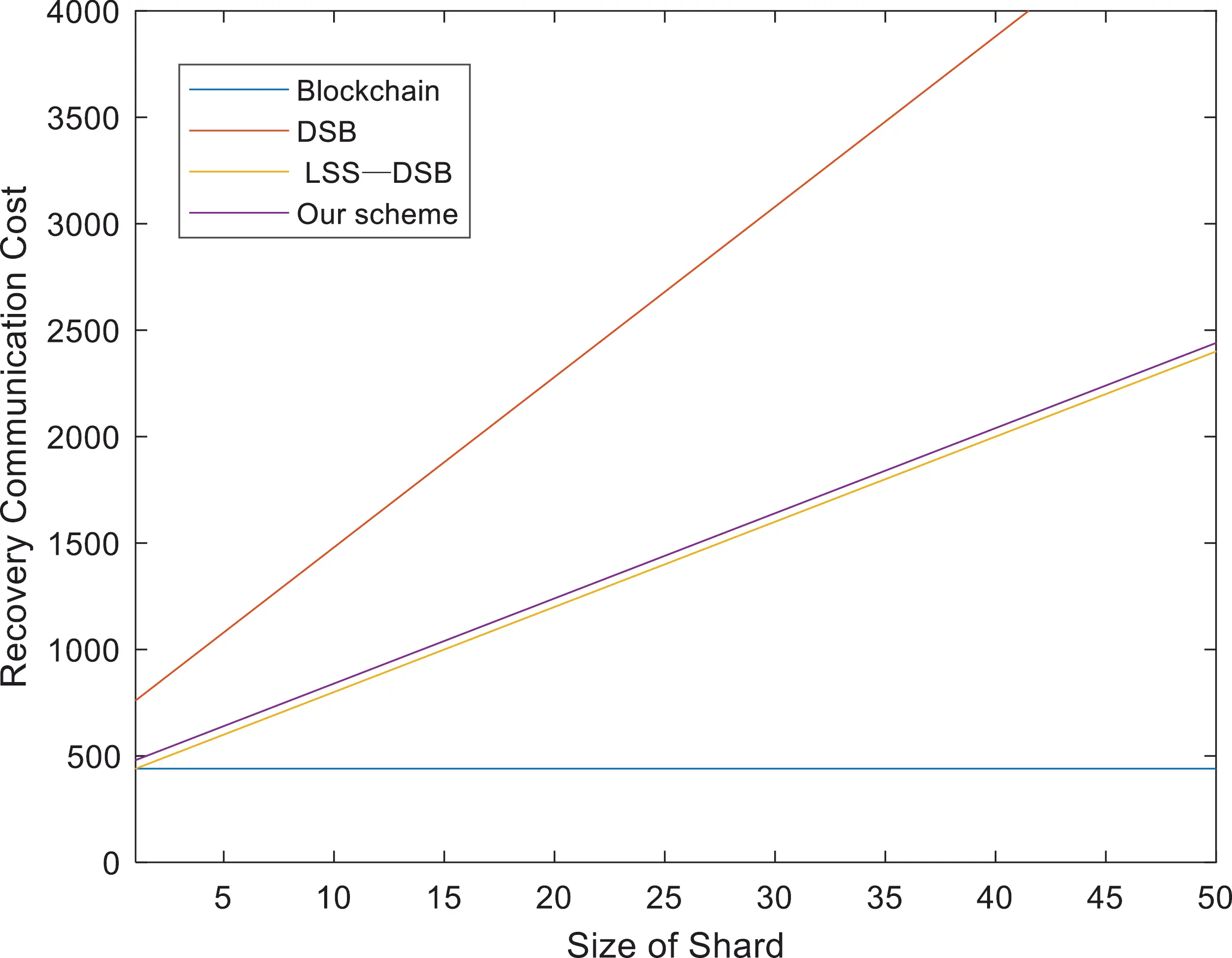Figure 4: Comparison of recovery communication cost

DSB. Different from traditional blockchain, DSB uses coding technology to reduce storage overhead, but the node needs to store a private key. The storage overhead for each node of DSB to store a transaction is:

StorDSB=log2τr+1+2log2p(20)

LSS-DSB. Local secret share (LSS) divides secrets into one global secret and many local secrets. The most important information will be treated as global secrets. The LSS-based DSB scheme can efficiently store private keys and hash values, which can further reduce storage overhead. The storage overhead for each node of LSS-DSB to store a transaction is:

StorLSSDSB=log2τr+log2p(21)

Our scheme. In our scheme, the node does not need to store additional private keys. The storage overhead of each node storing a transaction is:

StorPVSSDSB=log2τr+1+log2p(22)

Assumingp=2400, τ=240 and γ=200, the comparison of storage overhead is shown in Fig. 5. From the figure, we can see that as the size of shard increases, the storage cost of the blockchain is constant, and our scheme becomes smaller and tends to be constant as the size of the shard increases. Compared with several other schemes, our scheme is the best.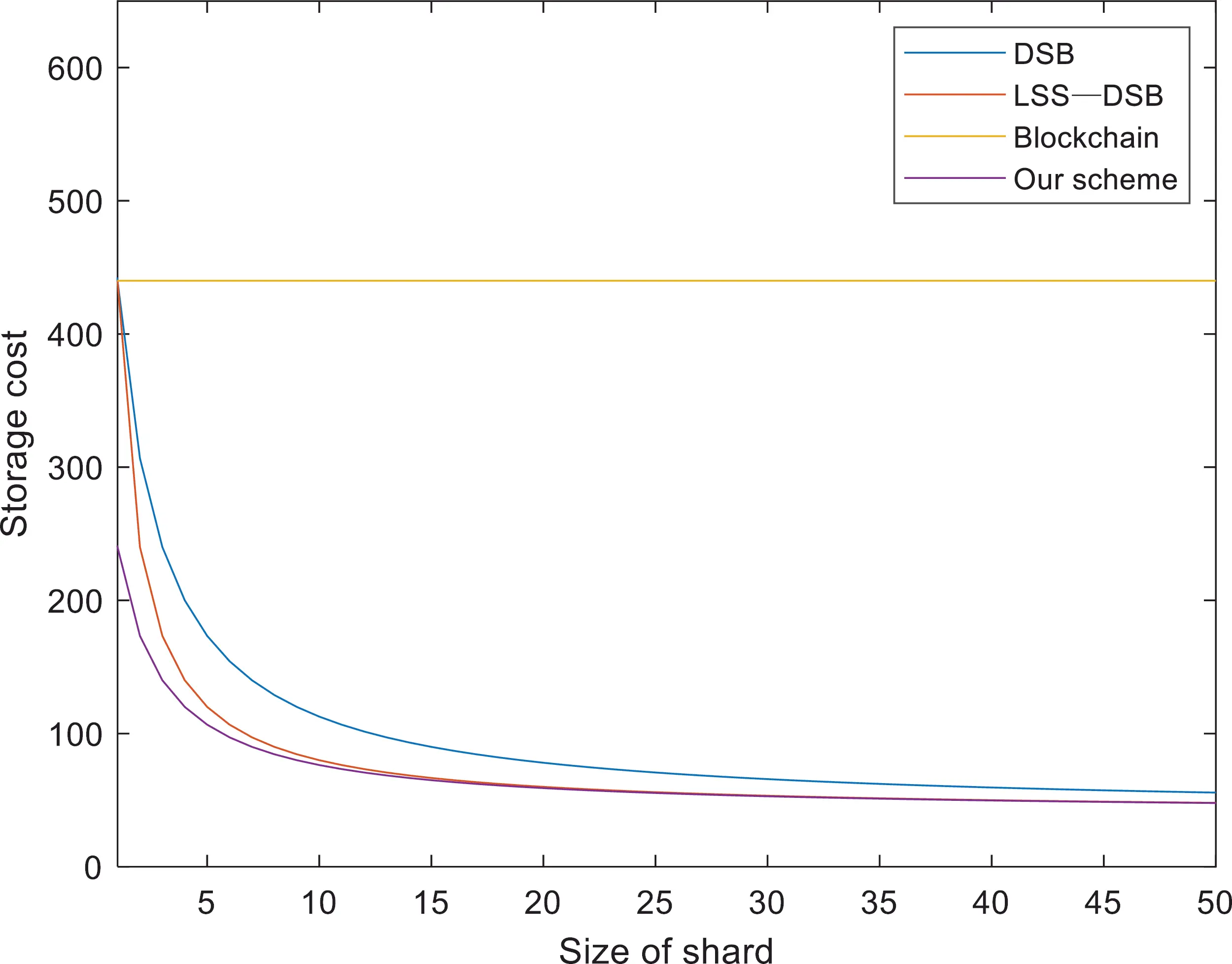Figure 5: Comparison of storage cost

10  Conclusion

Distributed storage is one of the important directions of future storage system development and blockchain provides solutions to the security and performance problems of distributed storage. This paper first uses polynomial commitment to improve verifiable secret share and constructs a new VSS scheme. Then use the new VSS scheme to construct a distributed storage mechanism based on blockchain. Compared with the previous scheme, the scheme proposed in this paper also achieves low storage cost, and is also superior to the DSB scheme in terms of recovery communication cost. Future research directions mainly include the following points: (1) Replace transaction and network with state sharding to further optimize storage cost; (2) Realize efficient communication across partitions.

Funding Statement: This work was supported by the National Natural Science Foundation of China under Grant 62072249, 61772280, 61772454, 62072056. J. Wang and Y. Ren received the grants, and the URL of the sponsors' website is http://www.nsfc.gov.cn/. This work was also supported by the Project of Transformation and Upgrading of Industries and Information Technologies of Jiangsu Province (No. JITC-1900AX2038/01). X. Yu received the grant, and the URL of the sponsors' website is http://gxt.jiangsu.gov.cn/.

Conflicts of Interest: The authors declare that they have no conflicts of interest to report regarding the present study.

## References

1. S. Rawat, D. S. Papailiopoulos, A. G. Dimakis and S. Vishwanath, “Locality and availability in distributed storage,” IEEE Transactions on Information Theory, vol. 62, no. 8, pp. 4448–4493, 2016.
2. L. Fang, Y. Li, X. Yun, Z. Wen, S. Ji et al., “THP: A novel authentication scheme to prevent multiple attacks in SDN-based IoT network,” IEEE Internet of Things, vol. 7, no. 7, pp. 5745–5759, 2020.
3. H. Yang, W. Shin and J. Lee, “Private information retrieval for secure distributed storage systems,” IEEE Transactions on Information Forensics and Security, vol. 13, no. 12, pp. 2953–2964, 2018.
4. Y. Ren, F. Zhu, P. K. Sharma, T. Wang, J. Wang et al., “Data query mechanism based on hash computing power of blockchain in internet of things,” Sensors, vol. 20, no. 1, pp. 1–20, 2020.
5. J. Wang, Y. Gao, W. Liu, A. K. Sangaiah and H. J. Kim, “An intelligent data gathering schema with data fusion supported for mobile sink in wireless sensor networks,” International Journal of Distributed Sensor Networks, vol. 15, no. 3, pp. 326–340, 2019.
6. J. Wang, C. Ju, Y. Gao, A. K. Sangaiah and G. J. Kim, “A PSO based energy efficient coverage control algorithm for wireless sensor networks,” Computers, Materials & Continua, vol. 56, no. 3, pp. 433–446, 2018.
7. Z. Zheng, S. Xie, H. N. Dai, X. Chen and H. Wang, “Blockchain challenges and opportunities: A survey,” International Journal of Web and Grid Services, vol. 14, no. 4, pp. 352, 2018.
8. J. Wang, Y. Yang, T. Wang, R. S. Sherratt and J. Zhang, “Big data service architecture: A survey,” Journal of Internet Technology, vol. 21, no. 2, pp. 393–405, 2020.
9. T. T. A. Dinh, R. Liu, M. Zhang, G. Chen, B. C. Ooi et al., “Untangling blockchain: A data processing view of blockchain systems,” IEEE Transactions on Knowledge and Data Engineering, vol. 30, no. 7, pp. 1366–1385, 2018.
10. C. Ge, Z. Liu, J. Xia and L. Fang, “Revocable identity-based broadcast proxy re-encryption for data sharing in clouds,” IEEE Transactions on Dependable and Secure Computing, vol. 18, no. 3, pp. 1214–1226, 2021.
11. S. Basu, A. Tomescu, I. Abraham, D. Malkhi, M. K. Reiter et al., “Efficient verifiable secret sharing with share recovery in BFT protocols,” in Proc. of the ACM Conf. on Computer and Communications Security, Hong Kong, HK, China, pp. 2387–2402, 2019.
12. A. N. Amroudi, A. Zaghain and M. Sajadieh, “A verifiable (k,n,m)-threshold multi-secret sharing scheme based on NTRU cryptosystem,” Wireless Personal Communications, vol. 96, no. 1, pp. 1393–1405, 2017.
13. L. Harn and C. Lin, “Strong (n, t, n) verifiable secret sharing scheme,” Information Sciences, vol. 180, no. 16, pp. 3059–3064, 2010.
14. Y. X. Liu, L. Harn, C. N. Yang and Y. Q. Zhang, “Efficient (n, t, n) secret sharing schemes,” Journal of Systems and Software, vol. 85, no. 6, pp. 1325–1332, 2012.
15. I. Miers, G. Christina, G. Matthew and A. D. Rubin, “Zerocoin: Anonymous distributed e-cash from bitcoin,” in Proc. 2013 IEEE Symp. on Security and Privacy, San Francisco, CA, USA, pp. 397–411, 2013.
16. A. Kate, G. M. Zaverucha and I. Goldberg, “Constant-size commitments to polynomials and their applications,” in Proc. ASIACRYPT 2010, Singapore, pp. 177–194, 2010.
17. H. Y. Paik, X. Xu, H. D. Bandara, S. U. Lee and S. K. Lo, “Analysis of data management in blockchain-based systems: From architecture to governance,” IEEE Access, vol. 7, pp. 186091–186107, 2019.
18. Y. Ren, Y. Leng, Y. Cheng and J. Wang, “Secure data storage based on blockchain and coding in edge computing,” Mathematical Biosciences and Engineering, vol. 16, no. 4, pp. 1874–92, 2019.
19. Y. Tian, Z. Wang, J. Xiong and J. Ma, “A blockchain-based secure key management scheme with trustworthiness in DWSNs,” IEEE Transactions on Industrial Informatics, vol. 16, no. 9, pp. 6193–6202, 2020.
20. H. Dang, T. T. A. Dinh, D. Loghin, E. C. Chang, Q. Lin et al., “Towards scaling blockchain systems via sharding,” in Proc. of the 2019 Int. Conf. on Management of Data, New York, NY, USA, pp. 123–140, 2019.
21. M. Zamani, M. Movahedi and M. Raykova, “Rapidchain: Scaling blockchain via full sharding,” in Proc. of the 2018 ACM SIGSAC Conf. on Computer and Communications Security, Toronto, Canada, pp. 931–948, 2018.
22. A. G. Dimakis, P. B. Godfrey, Y. Wu, M. J. Wainwright and K. Ramchandran, “Network coding for distributed storage systems,” IEEE Transactions on Information Theory, vol. 56, no. 9, pp. 4539–4551, 2007.
23. J. Wang, Y. Gao, W. Liu, W. Wu and S. J. Lim, “An asynchronous clustering and mobile data gathering schema based on timer mechanism in wireless sensor networks,” Computers, Materials & Continua, vol. 58, no. 3, pp. 711–725, 2019.
24. J. Wang, X. Gu, W. Liu, A. K. Sangaiah and H. J. Kim, “An empower hamilton loop-based data collection algorithm with mobile agent for WSNs,” Human-centric Computing and Information Sciences, vol. 9, no. 1, pp. 1–14, 2019.
25. M. Backes, A. Datta and A. Kate, “Asynchronous computational VSS with reduced communication complexity,” in Proc. Cryptographers’ Track at the RSA Conf., San Francisco, CA, USA, pp. 259–276, 2013.
26. C. P. Ge, W. Susilo, J. Baek, Z. Liu, J. Xia et al, “A verifiable and fair attribute-based proxy re-encryption scheme for data sharing in clouds,” IEEE Transactions on Dependable and Secure Computing, vol. 5972, no. c, pp. 1, 2021.
27. J. Wang, W. Wu, Z. Liao, R. S. Sherratt, G. J. Kim et al, “A probability preferred priori offloading mechanism in mobile edge computing,” IEEE Access, vol. 8, pp. 39758–39767, 2020.
28. M. Sheikhi-Garjan, M. Bahramian and C. Doche, “Threshold verifiable multi-secret sharing based on elliptic curves and Chinese remainder theorem,” IET Information Security, vol. 13, no. 3, pp. 278–284, 2019.
29. L. J. Pang and Y. M. Wang, “A new (t, n) multi-secret sharing scheme based on shamir's secret sharing,” Applied Mathematics and Computation, vol. 167, no. 2, pp. 840–848, 2005.
30. J. Zhao, J. Zhang and R. Zhao, “A practical verifiable multi-secret sharing scheme,” Computer Standards & Interfaces, vol. 29, no. 1, pp. 138–141, 2007.
31. Z. Eslami and J. Z. Ahmadabadi, “A verifiable multi-secret sharing scheme based on cellular automata,” Information Sciences, vol. 180, no. 15, pp. 2889–2894, 2010.
32. N. Wang, Y. Cai, J. Fu and X. Chen, “Information privacy protection based on verifiable (t, n)-threshold multi-secret sharing scheme,” IEEE Access, vol. 8, pp. 20799–20804, 2020.
33. H. Zhang, P. Cheng, L. Shi and J. Chen, “Optimal DoS attack scheduling in wireless networked control system,” IEEE Transactions on Control Systems Technology, vol. 24, no. 3, pp. 843–852, 2015.
34. C. Ge, W. Susilo, Z. Liu, J. Xia, P. Szalachowski et al., “Secure keyword search and data sharing mechanism for cloud computing,” IEEE Transactions on Dependable and Secure Computing, vol. 5971, no. c, pp. 1, 2020.
35. J. Xiong, R. Bi, M. Zhao, J. Guo and Q. Yang, “Edge-assisted privacy-preserving raw data sharing framework for connected autonomous vehicles,” IEEE Wireless Communications, vol. 27, no. 3, pp. 24–30, 2020.
36. J. Y. Ren, J. Qi, Y. Cheng, J. Wang and A. Osama, “Digital continuity guarantee approach of electronic record based on data quality theory,” Computers, Materials & Continua, vol. 63, no. 3, pp. 1471–1483, 2020.
37. J. Wang, Y. Zou, P. Lei, R. S. Sherratt and L. Wang, “Research on recurrent neural network-based crack opening prediction of concrete dam,” Journal of Internet Technology, vol. 21, no. 4, pp. 1161–1169, 2020.
38. C. P. Ge, W. Susilo, J. Baek, Z. Liu, J. Xia et al., “Revocable attribute-based encryption with data integrity in clouds,” IEEE Transactions on Dependable and Secure Computing, vol. 5972, no. c, pp. 1, 2021.
39. J. Wang, Y. Gao, C. Zhou, S. Sherratt and L. Wang, “Optimal coverage multi-path scheduling scheme with multiple mobile sinks for WSNs,” Computers, Materials & Continua, vol. 62, no. 2, pp. 695–711, 2020.
40. G. Xu, X. Li, L. Jiao, W. Wang, A. Liu et al., “BAGKD: A batch authentication and group key distribution protocol for VANETs,” IEEE Communications Magazine, vol. 58, no. 7, pp. 35–41, 2020.
41. Y. Ren, J. Qi, Y. Liu, J. Wang and G. Kim, “Integrity verification mechanism of sensor data based on bilinear map accumulator,” ACM Transactions on Internet Technology, vol. 21, no. 1, pp. 1–19, 2021.
42. R. K. Raman and L. R. Varshney, “Distributed storage meets secret sharing on the blockchain,” in Proc. 2018 Information Theory and Applications Workshop (ITASan Francisco, CA, USA, pp. 1–6, 2018.
43. Y. Ren, Y. Leng, J. Qi, P. K. Sharma, J. Wang et al., “Multiple cloud storage mechanism based on blockchain in smart homes,” Future Generation Computer Systems, vol. 115, pp. 304–313, 2021.
44. Y. Kim, R. K. Raman, Y. S. Kim, L. R. Varshney and N. R. Shanbhag, “Efficient local secret sharing for distributed blockchain systems,” IEEE Communications Letters, vol. 23, no. 2, pp. 282–285, 2018.
45. J. Wang, Y. Tang, S. He, C. Zhao, P. K. Sharma et al., “Logevent2vec: LogEvent-to-vector based anomaly detection for large-scale logs in internet of things,” Sensors, vol. 20, no. 9, pp. 1–19, 2020.This work is licensed under a Creative Commons Attribution 4.0 International License, which permits unrestricted use, distribution, and reproduction in any medium, provided the original work is properly cited.